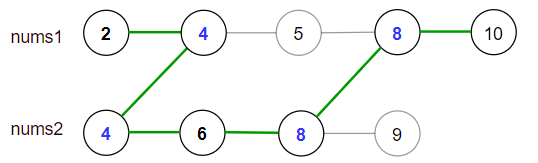# 最大得分

## 1537. 最大得分 (Hard)

• 选择数组 nums1 或者 nums2 开始遍历（从下标 0 处开始）。
• 从左到右遍历当前数组。
• 如果你遇到了 `nums1` 和 `nums2` 中都存在的值，那么你可以切换路径到另一个数组对应数字处继续遍历（但在合法路径中重复数字只会被统计一次）。```输入：nums1 = [2,4,5,8,10], nums2 = [4,6,8,9]

[2,4,5,8,10], [2,4,5,8,9], [2,4,6,8,9], [2,4,6,8,10],（从 nums1 开始遍历）
[4,6,8,9], [4,5,8,10], [4,5,8,9], [4,6,8,10]  （从 nums2 开始遍历）

```

```输入：nums1 = [1,3,5,7,9], nums2 = [3,5,100]

```

```输入：nums1 = [1,2,3,4,5], nums2 = [6,7,8,9,10]

```

```输入：nums1 = [1,4,5,8,9,11,19], nums2 = [2,3,4,11,12]

```

• `1 <= nums1.length <= 10^5`
• `1 <= nums2.length <= 10^5`
• `1 <= nums1[i], nums2[i] <= 10^7`
• `nums1` 和 `nums2` 都是严格递增的数组。

[动态规划]